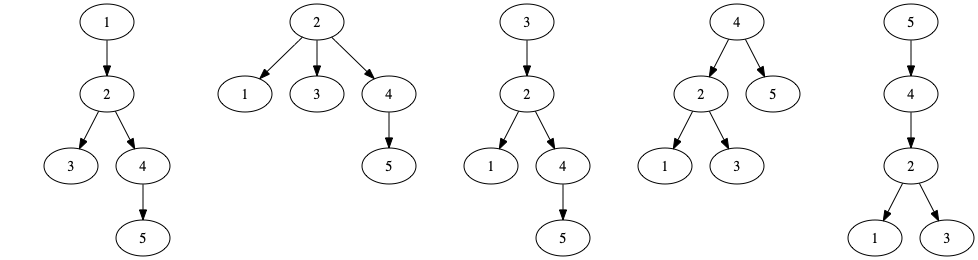시간 제한메모리 제한제출정답맞은 사람정답 비율
11 초 (추가 시간 없음) 512 MB41272165.625%

## 문제

A tree is a connected, acyclic, undirected graph with n nodes and n − 1 edges. There is exactly one path between any pair of nodes. A rooted tree is a tree with a particular node selected as the root.

Let T be a tree and Tr be that tree rooted at node r. The subtree of u in Tr is the set of all nodes v where the path from r to v contains u (including u itself). In this problem, we denote the set of nodes in the subtree of u in the tree rooted at r as Tr(u).

You are given q queries. Each query consists of two (not necessarily different) nodes, r and p. A set S is “obtainable” if and only if it can be expressed as the intersection of a subtree in the tree rooted at r and a subtree in the tree rooted at p. Formally, a set S is “obtainable” if and only if there exist nodes u and v where S = Tr(u) ∩ Tp(v).

For a given pair of roots, count the number of different non-empty obtainable sets. Two sets are different if and only if there is an element that appears in one, but not the other.

## 입력

The first line contains two space-separated integers n and q (1 ≤ n, q ≤ 2 · 105), where n is the number of nodes in the tree and q is the number of queries to be answered. The nodes are numbered from 1 to n.

Each of the next n − 1 lines contains two space-separated integers u and v (1 ≤ u, v ≤ n, u ≠ v), indicating an undirected edge between nodes u and v. It is guaranteed that this set of edges forms a valid tree.

Each of the next q lines contains two space-separated integers r and p (1 ≤ r, p ≤ n), which are the nodes of the roots for the given query.

## 출력

For each query output a single integer, which is the number of distinct obtainable sets of nodes that can be generated by the above procedure.

## 예제 입력 1

5 2
1 2
2 3
2 4
4 5
1 3
4 5


## 예제 출력 1

8
6


## 힌트

The possible rootings of the first tree areConsidering the rootings at 1 and 3, the 8 obtainable sets are:

1. {1} by choosing u = 1, v = 1,
2. {1, 2, 4, 5} by choosing u = 1, v = 2,
3. {1, 2, 3, 4, 5} by choosing u = 1, v = 3,
4. {2, 3, 4, 5} by choosing u = 2, v = 3,
5. {2, 4, 5} by choosing u = 2, v = 2,
6. {3} by choosing u = 3, v = 3,
7. {4, 5} by choosing u = 2, v = 4,
8. and {5} by choosing u = 5, v = 5.

If the rootings are instead at 4 and 5, there are only 6 obtainable sets:

1. {1} by choosing u = 1, v = 1,
2. {1, 2, 3} by choosing u = 2, v = 4,
3. {1, 2, 3, 4} by choosing u = 4, v = 4,
4. {1, 2, 3, 4, 5} by choosing u = 4, v = 5,
5. {3} by choosing u = 3, v = 2,
6. and {5} by choosing u = 5, v = 5.

For some of these, there are other ways to choose u and v to arrive at the same set.